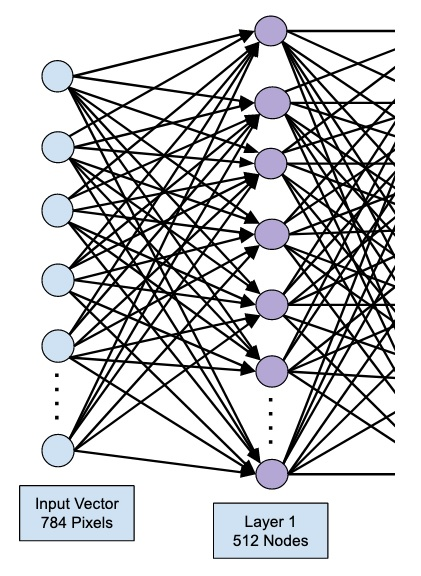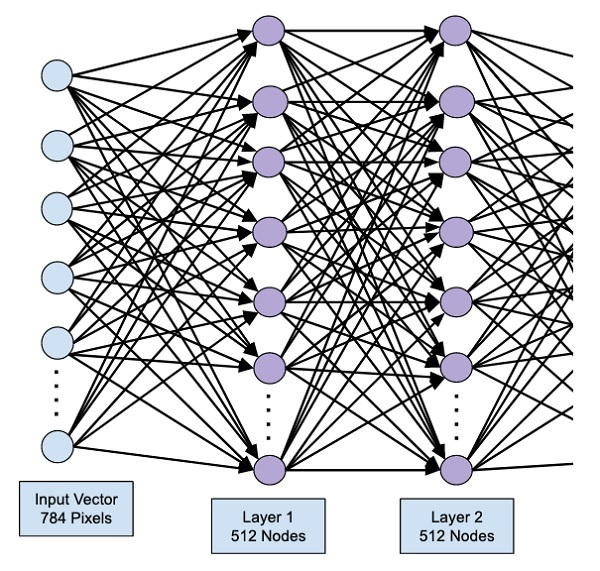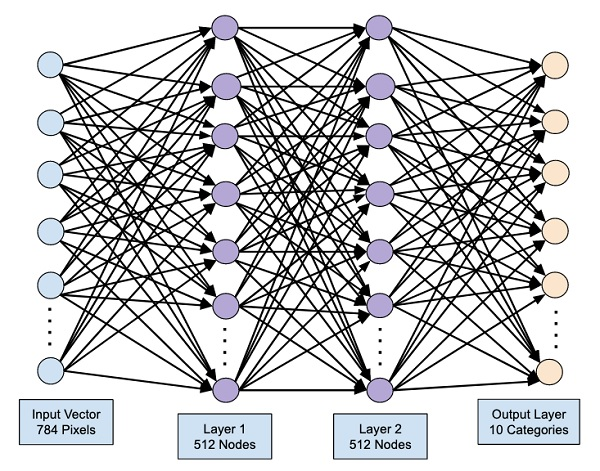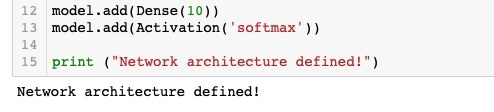# Creating Deep Learning Model

Our neural network model will consist of a linear stack of layers. To define such a model, we call the Sequential function −

```model = Sequential()
```

## Input Layer

We define the input layer, which is the first layer in our network using the following program statement −

```model.add(Dense(512, input_shape=(784,)))
```

This creates a layer with 512 nodes (neurons) with 784 input nodes. This is depicted in the figure below −Note that all the input nodes are fully connected to the Layer 1, that is each input node is connected to all 512 nodes of Layer 1.

Next, we need to add the activation function for the output of Layer 1. We will use ReLU as our activation. The activation function is added using the following program statement −

```model.add(Activation('relu'))
```

Next, we add Dropout of 20% using the statement below. Dropout is a technique used to prevent model from overfitting.

```model.add(Dropout(0.2))
```

At this point, our input layer is fully defined. Next, we will add a hidden layer.

## Hidden Layer

Our hidden layer will consist of 512 nodes. The input to the hidden layer comes from our previously defined input layer. All the nodes are fully connected as in the earlier case. The output of the hidden layer will go to the next layer in the network, which is going to be our final and output layer. We will use the same ReLU activation as for the previous layer and a dropout of 20%. The code for adding this layer is given here −

```model.add(Dense(512))
```

The network at this stage can be visualized as follows −Next, we will add the final layer to our network, which is the output layer. Note that you may add any number of hidden layers using the code similar to the one which you have used here. Adding more layers would make the network complex for training; however, giving a definite advantage of better results in many cases though not all.

## Output Layer

The output layer consists of just 10 nodes as we want to classify the given images in 10 distinct digits. We add this layer, using the following statement −

```model.add(Dense(10))
```

As we want to classify the output in 10 distinct units, we use the softmax activation. In case of ReLU, the output is binary. We add the activation using the following statement −

```model.add(Activation('softmax'))
```

At this point, our network can be visualized as shown in the below diagram −At this point, our network model is fully defined in the software. Run the code cell and if there are no errors, you will get a confirmation message on the screen as shown in the screenshot below −Next, we need to compile the model.